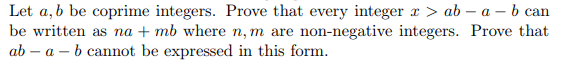# Let a, b be coprime integers. Prove that every integer x > ab- a- b canbe written as na mb where n, m are non-negative integers. Prove thatab -a - b cannot be expressed in this form

Question
12 views

Abstract Algebrahelp_outlineImage TranscriptioncloseLet a, b be coprime integers. Prove that every integer x > ab- a- b can be written as na mb where n, m are non-negative integers. Prove that ab -a - b cannot be expressed in this form fullscreen
check_circle

Step 1

To find non-negative representations under the given conditions

Step 2

In the following, a and b are coprime positive integers; Note that , by Euclidean algorithm, ANY integer x is an integral linear combination na+mb. The point is we seek sufficient condtions on  the integer x ( namely x>ab-a-b) na+nb=x with both n and m non-negative,For instance , consider a =10, b =3, clearly relatively prime positive integers . Now x=10+3=13 is a non-negative integral linear combination of a and b , but 14,16, 17 are not. The theorem asserts that whenver x > 10*3-10-3=17,x can be expressed as 10n+3m , with n and m non-negative integers.

Step 3

General solution of (1) is derived , using the ...

### Want to see the full answer?

See Solution

#### Want to see this answer and more?

Solutions are written by subject experts who are available 24/7. Questions are typically answered within 1 hour.*

See Solution
*Response times may vary by subject and question.
Tagged in

### Math